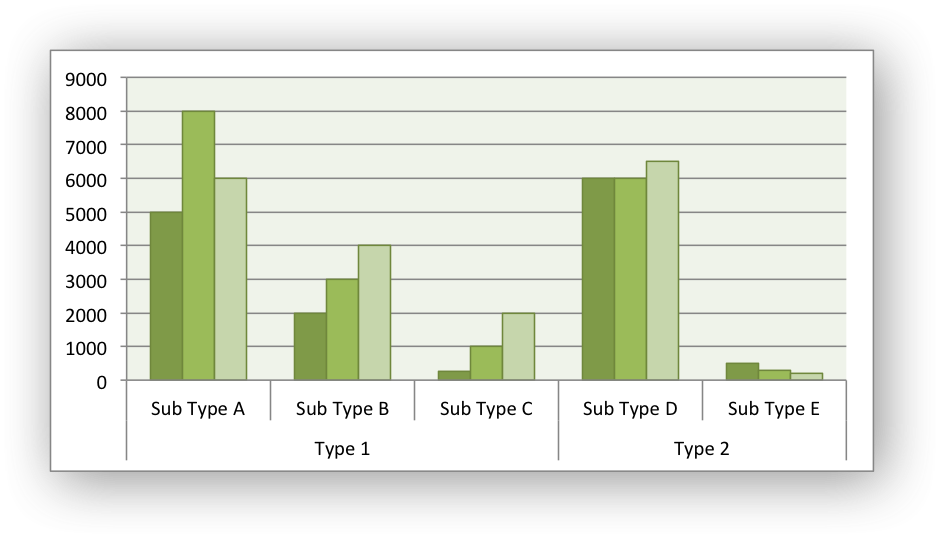# Example: Clustered Chart#

Example of creating a clustered Excel chart where there are two levels of category on the X axis.The categories in clustered charts are 2D ranges, instead of the more normal 1D ranges. The series are shown as formula strings for clarity but you can also use the a list syntax.

```#######################################################################
#
# A demo of a clustered category chart in XlsxWriter.
#
# Copyright 2013-2023, John McNamara, jmcnamara@cpan.org
#
from xlsxwriter.workbook import Workbook

workbook = Workbook("chart_clustered.xlsx")

# Add the worksheet data that the charts will refer to.
headings = ["Types", "Sub Type", "Value 1", "Value 2", "Value 3"]
data = [
["Type 1", "Sub Type A", 5000, 8000, 6000],
["", "Sub Type B", 2000, 3000, 4000],
["", "Sub Type C", 250, 1000, 2000],
["Type 2", "Sub Type D", 6000, 6000, 6500],
["", "Sub Type E", 500, 300, 200],
]

for row_num, row_data in enumerate(data):
worksheet.write_row(row_num + 1, 0, row_data)

# Create a new chart object. In this case an embedded chart.

# Configure the series. Note, that the categories are 2D ranges (from column A
# to column B). This creates the clusters. The series are shown as formula
# strings for clarity but you can also use the list syntax. See the docs.
{
"categories": "=Sheet1!\$A\$2:\$B\$6",
"values": "=Sheet1!\$C\$2:\$C\$6",
}
)

{
"categories": "=Sheet1!\$A\$2:\$B\$6",
"values": "=Sheet1!\$D\$2:\$D\$6",
}
)

{
"categories": "=Sheet1!\$A\$2:\$B\$6",
"values": "=Sheet1!\$E\$2:\$E\$6",
}
)

# Set the Excel chart style.
chart.set_style(37)

# Turn off the legend.
chart.set_legend({"position": "none"})

# Insert the chart into the worksheet.
worksheet.insert_chart("G3", chart)

workbook.close()
```